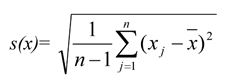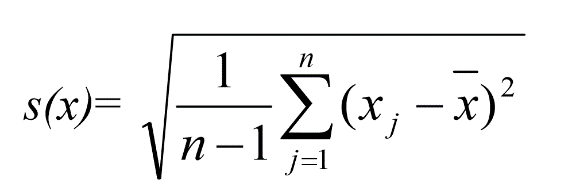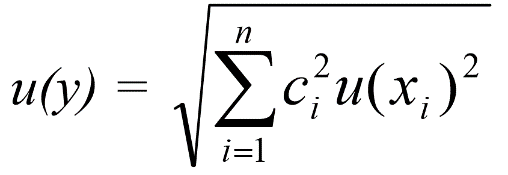by Perri Zilberman

Intro

What is measurement uncertainty?

According to the International Vocabulary of Metrology – Basic and General Concepts and Associated Terms (VIM) 3rd edition, prepared by the Joint Committee for Guides in Metrology, measurement uncertainty is defined to be a “non-negative parameter characterizing the dispersion of the quantity values being attributed to a measurand, based on the information used.” Here, a quantity value is defined to be a “number and reference together expressing magnitude of a quantity,” and a measurand is defined to be the “quantity intended to be measured.” In layman’s terms, measurement uncertainty can be said to be how precise a measurement is, or alternatively, how confident we can be that a measurement is a certain value. Here, precision is defined to be “the closeness of agreement between independent measurements of a quantity under the same conditions.” (NDT Resource Center). Precision should not be confused with accuracy, which is “the closeness of agreement between a measured value and the true value.” (NDT Resource Center).

The “Guide to the Expression of Uncertainty in Measurement,” or GUM for short, is the generally accepted guide to all things measurement uncertainty related.

Why is measurement uncertainty important?

No measurement is free from uncertainty. The reasons for why this is are explored in the next section. Nonetheless, industry leaders and researchers still often desire microspheres of only one very specific size. Understanding and knowing how to limit measurement uncertainty is key to reconciling these two competing factors.

Where does measurement uncertainty come from?

All measurements are subject to measurement uncertainty. This is for the simple reason that one cannot be infinitely precise or accurate. Take for example, someone attempting to measure a pencil using a ruler. Simple enough. All one would have to do would be to lie the pencil next to the ruler and see where the pencil falls with regard to the measurement marks. However, perhaps the pencil falls above or below a certain mark. Perhaps the marks are not spaced perfectly apart. Even if the ruler is perfect (which is, of course, impossible) and the pencil is also happens to be the exact height as one of the denominations on the ruler (which is also, again, impossible), human eyes have a finite resolution! Perhaps this perfect pencil is exactly 19 cm, and the perfect ruler shows this. Assuming all one has is the pencil, the ruler, and their eyes, how would one know that the pencil is exactly 19 cm, and not, say, 19.0001 cm? or 18.9999 cm? Of course, one can’t. A pencil that is exactly 19 cm would be completely indistinguishable from one that is 19.0001 cm or 18.9999 cm, using only human eyes. One simply can’t be 100% confident that our perfectly 19 cm tall pencil is actually perfectly 19 cm tall. However what one can do is say that the pencil appears to be 19 cm tall plus and minus x amount, with x being the difference between a pencil height that is distinguishable from 19 cm, and 19 cm. This is what measurement uncertainty essentially boils down to: how confident one is in a measurement.

Why can’t one simply use a more precise device?

One can! However, this wouldn’t eliminate measurement uncertainty. It would simply decrease it. Continuing with the perfect pencil and perfect ruler example from the previous paragraph, one can use a standard optical microscope and see that our perfectly 19 cm tall pencil is extremely close to 19 cm. Much closer than can see with their naked eyes. However, we come again to the same problem. Using this optical microscope, how would one know that our perfect pencil isn’t actually 19.000001 cm or 18.999999 cm? After all, optical microscopes have resolution limits as well. Even an electron microscope, perhaps the most precise instrument created by mankind has a resolution limit. All measurements are subject to uncertainty.

How does measurement uncertainty work on the microscopic scale?

Broadly speaking, measurement uncertainty works much the same way on the microscopic scale as it does on the macroscopic scale. After all, one is still just taking measurements. However, performing precise measurements on the microscopic level presents several more areas in which measurement uncertainty occurs. These must be understood and taken into account to obtain the best possible measurements.

For one, all optical microscopes are limited to a maximum resolution of around 200nm. This is derived from the Rayleigh Criterion, a formula describing the maximum resolvable detail through an instrument. In our case, the Rayleigh Criterion can be expressed as:

d=0.61Λ/NA

Here, d is the maximum possible resolution, Λ is the wavelength of light that you are working with, and NA is the objective numerical aperture. The objective numerical aperture is defined by the function:

NA=n*sin(µ)

Where NA is the objective numerical aperture, n is the index of refraction of the imaging medium between the objective and measurand, and µ is one half of the angular aperture. Assuming an imaging medium of air, it is hard to obtain an objective numerical aperture larger than 0.95. The wavelength of visible light ranges from 390 to 700nm. Once these are put into the Rayleigh Criterion formula, one sees that the smallest resolvable detail for an optical microscope is from ~250 – 449nm, depending on the wavelength of light. It can be higher, however, if one uses an imaging medium with a higher index of refraction than air, such as oil. Even then, assuming one uses an imaging medium of immersion oil with an index of refraction of 1.51, the maximum resolvable detail using an optical microscope only falls to ~166 – 298nm, again depending upon the wavelength of light used. This maximum resolution, however, assumes a perfect measurement tool. No such tool exists, unfortunately, so the effective resolution of a microscope will always be worse than 0.2 microns (micro.magnet.fsu.edu).

Object focus, of course, is also an issue. After all, improper focusing may not only change the apparent size of the measurand, but will likely create slightly blurred edges, making performing an accurate measurement of the object difficult. Especially since many laboratories focus using humans, as opposed to a dedicated focusing to.

Further, optical microscopes are limited to only imaging dark objects or objects with a large index of refraction. This is due to the fact that one needs to be able to distinguish the object that one is measuring from its surroundings. This necessitates the measurand having a sufficient contrast from its surroundings. If in object is extremely light (and no staining is performed) then that needed contrast will not be there. It is the same story for a large index of refraction; How can one measure a microsphere if it is completely transparent? This need for a large contrast between the object being measured and the medium it is being measured in also necessitates the need for proper lighting. One will have quite a difficult time making out a microsphere well if the image is too dark or too bright.

Beyond simply the limitations of optical microscopes, one must realize that when measuring tiny objects such as microspheres in a laboratory setting, tools such as microscope cameras and image analysis software is also used. These present their own slew of areas in which measurement uncertainty must be taken into account.

The microscope camera, for instance, has its own finite resolution, imperfect optics, and an imperfect sensor. Each of these affects how precisely one can measure an object. Imperfect optics may blur the edges of the object and an imperfect sensor might not pick up on the contrast that the measurand has compared with its surroundings. Likewise, one must also take into account the uncertainty associated with any image software analysis used. Perhaps the software has a difficult time making out the microsphere from its surroundings, or the software isn’t designed to work with microspheres past a certain size. These must all be taken into account.

Of course, the impact of each of these factors will depend on the equipment and software that is used. If one does not focus well, for instance, that would likely create an uncertainty far larger than the resolution of the microscope would. Or if the image analysis software used is extremely poor, then that would likely introduce a much larger uncertainty than the imperfect optics of the microscope would (assuming that the optics are of high quality, and properly calibrated). One should attempt to minimize the uncertainty of all of these factors.

How is measurement uncertainty calculated?

Please note that all equations in this section are taken from Uncertainty and Confidence in Measurement by Ioannis Kechagiogou.

Measurement uncertainty can be calculated in several different ways. As was previously mentioned, measurement uncertainty is essentially how confidant one is in a measurement. If one is using a somewhat rudimentary measuring technique (such as “eyeballing it”), the measurement uncertainty rests on the expertise and judgement of the experimenter. Continuing with the pencil and ruler analogy, let us say that one is measuring a pencil that is just a tad longer than 19 cm, using a meterstick with 1mm markings. One can confidently say that the pencil falls between 19 cm and 19.1 cm. Perhaps it looks like the pencil falls halfway between the two markings, but the experimenter isn’t sure. He’s sure that the pencil isn’t 19.02 cm, and he knows for certain that it isn’t 19.08 cm. The experimenter might then also take into account other possible areas of uncertainty, such as the uncertainty of how true the markings on the ruler are. There is, after all, the possibility that their markings aren’t perfectly spaced apart. He may then say that the pencil looks to be 19.05 ± 0.4 cm. As one might expect though, this does not produce entirely accurate measurement uncertainties.

When discussing measurement uncertainty in more depth, it must be acknowledged that there are two main types of measurement uncertainty: Type A, and type B. Type A encompasses “random” uncertainty. These are uncertainties that appear when repeating the same measurement multiple times. For example, one may measure the diameter of a microsphere using dial calipers and, all things still being equal throughout these measurements, obtain slightly different values for each measurement. Type B uncertainties, on the other hand can be considered “systematic” uncertainties. Type B uncertainties are essentially all uncertainties that aren’t type A. Type B uncertainties include but aren’t limited to: the resolution of a measurement device, calibration data, measurement device specifications, and knowledge and experience with the measurand and measurement device. Within the context of microscopy, type B uncertainties can be considered the uncertainties associated with imperfect focusing, imperfect image analysis software, and the resolution of both the microscope and the microscope camera. These two error types are dealt with in different ways. Afterwards, they are combined into a final measurement uncertainty value

In order to quantify type A uncertainty, one can perform several different measurements of the same object. From this, one can obtain a distribution of measurements. This distribution can assist in obtaining a probability distribution, which can, in turn, assist in deriving a confidence limit and standard deviation for the measurements. When plotted, these measurement distributions tend to be normal. Both of these can then be used in obtaining a measurement uncertainty. As one might also expect, measuring the same object multiple times and then obtaining the average also increases the accuracy of a measurement. If one is measuring every object in a population, one can use the equation:With Θ being the standard deviation, N being the number of elements in the entire population, and x¯ being the mean of the set. One can also input the information into a program such as Microsoft Excel or a sufficiently advanced calculator and calculate it using the standard deviation tool. In the case of Excel, the formula for calculating the standard deviation of a set is “=STDEV().”

However, in the much more likely scenario in where one is only measuring a sample of an entire population, one must use the formula:s(x) represents the estimate of the standard deviation of the entire population and n is the number of elements in your set (not the entire population). All other variables are the same.

Quantifying type B occurs in a somewhat different way compared to type A. In comparison to the normal distribution of measurements caused by type A uncertainties, a measurement distribution due to type B uncertainties often takes the form of a rectangular or triangular distribution, with the distribution width upper and lower limits being estimated by the experimenter. This is due to the fact the type B distributions create errors that tend to remain constant throughout all measurements. A rectangular distribution is used if it is believed that it is equally probable for the uncertainty value to fall anywhere between the upper and lower limits. This is often used as the default distribution for type B uncertainties. A standard deviation can then be obtained from the type B distribution In order to find the standard deviation of a rectangular distribution, one must use the equation:

s =a/√3

With s being the standard deviation, and a being the limits of the rectangular distribution. A triangular distribution should be used if it is believed that the uncertainty value is more likely to fall in the middle of the upper and lower limits and less likely to fall near each limit. To obtain the standard deviation of a triangular distribution, one must use the equation:

s =a/√6

With all variables being the same.

Afterwards, these uncertainties should be added together. Before one can do this, however, one must first convert all of uncertainties previously measured into standard deviations. For the following steps, standard uncertainty is used. This is essentially one standard deviation in a measurement uncertainty distribution. Afterwards, one can then combine all standard uncertainties utilizing the law of propagation of uncertainty. That is, one must use this equation:Here, u(y) is what is known as the “combined standard uncertainty,” ci is a sensitivity coefficient, and xi is an uncertainty component.

Next, one must then determine how confident one can be in this combined uncertainty. To do this, one must calculate what is known as the expanded uncertainty. This can be done through using the formula:

U=k*u(y)

With U being the expanded uncertainty, u(y) being the combined uncertainty, and k being a constant known as the coverage factor. The coverage factor essentially depends upon how often you would like measurements to fall within your uncertainty interval. A k of 2 or 3 is most common, with it representing 95% or 99% of measurements falling within your measurement uncertainty, respectively. This is the last step.

How does this apply to Cospheric?

Cospheric prides itself on having the most precise microspheres on the market. However, despite our production and quality control tools being top of the line, and our materials being the best on the market, they are still imperfect human constructions. As such, they suffer the same problems as were outlined previously: they cannot be infinitely precise. When we measure our spheres, whether it be for quality control or production, there comes a certain degree of precision can simply not be exceeded. It should be noted though that this applies to all companies, not simply Cospheric. This limitation, within this specific context, comes from several different factors some of which can be controlled and some which cannot.

One of the largest factors of measurement uncertainty is the number of steps taken when measuring a sample. Indeed, due to the microscopic size of most of our products (often being on the order of mist droplets or cell organelles), our measurement process requires several more steps than using a ruler to measure a pencil. This is because each individual step in the measuring process has its own measurement uncertainty, and these uncertainties must all be added together to obtain the final measurement uncertainty. Further, each individual step that Cospheric takes when measuring a microsphere is itself sophisticated, meaning that each step in the measuring process has several areas in which uncertainties must be considered. For example, let us look at the first step measuring a microsphere: looking at them through a microscope. As already stated, microscopes themselves have a resolution limit, meaning that they cannot be infinitely precise. In addition to this, we must also properly focus on the microspheres and use the appropriate lighting. These all add to the measurement uncertainty. Even the type of microsphere that is being measured has a large effect on the measurement uncertainty. How well a microsphere’s edges are seen through an optical microscope depends partly on the type of microsphere. Some microspheres are prone to producing an “aura” under an optical microscope under improper lighting. Others may produce a glare under improper lighting, making it difficult to discern their edge through an optical microscope.

For this reason, we cannot say with 100% confidence that all our spheres are of one exact size. This is why we advertise our products as having a range of possible sizes (or alternatively, centering around a particular size combined with a tolerance).

How does this affect me?

Although it would be wonderful if one could purchase microspheres of exactly one size, that’s simply not possible. Fortunately, microspheres of exactly one size are almost never needed. Obtaining microspheres within an appropriately small range of sizes or of an appropriately small size tolerance is usually all that is required. If you would like assistance in determining the proper size distribution or size tolerance of the microspheres you need, please don’t hesitate to ask one of Cospheric’s microsphere experts for assistance.

Sources:

Uncertainty and Confidence in Measurement, Ioannis Kechagioglou

International vocabulary of metrology – Basic and general concepts and associated terms (VIM) 3rd edition, JCGM

Evaluation of measurement data — Guide to the expression of uncertainty in measurement, JCGM

Products 0-0 of 0
Show